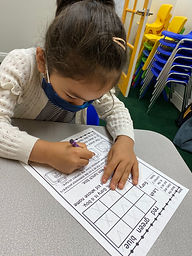Ms. Brianne

Target 1​

Lesson Type:

Continuation

Critical Thinking

:

Logical Reasoning

Use reasoning to solve logic puzzles.

1:

Use the given information in a problem to identify the correct answer.

2:

Use the given information to determine which is not the correct answer.

3:

Think outside of the box to solve problems.

4:

Follow given rules to solve problems.

2nd

Vocabulary:

logic puzzle, clue,

Activities:

Student

• solved logic puzzles using clues and the process of elimination.
• completed the Tower of Hanoi using three, four, and five discs.Home Exploration

Guiding Questions:Absent Students:

Target 2

:

2:

Set the foundation for later exploration of multiplication (i.e. 2+2+2+2 =? How many 2s did you add? When you add 4 number 2s together, what do you get?)

3:

Use addition to find the total number of objects in an array.

2nd

Vocabulary:

repeated addition, multiplication, array, skip counting

Activities:

Student

• wrote a multiplication and repeated addition equation when given a picture of fish in an array.
• used repeated addition to solve multiplication problems.
• noticed the pattern of skip counting when doing repeated additionHome Exploration

Guiding Questions:Target 3

:

Vocabulary:

Activities:Home Exploration